# Documentation for Binary Trees and Algorithms

We have a very detail documentation for Binary Trees and Algorithms with 4 sections, 15 sub sections, 25 images, 3 paragraphs and 4173 words

## Introduction

### Definition

Tree represents the nodes connected by edges. Each nodes may have or not have child nodes. A binary tree is a tree that each node can have a maximum of two children. The following figure shows the basic elements of a binary tree.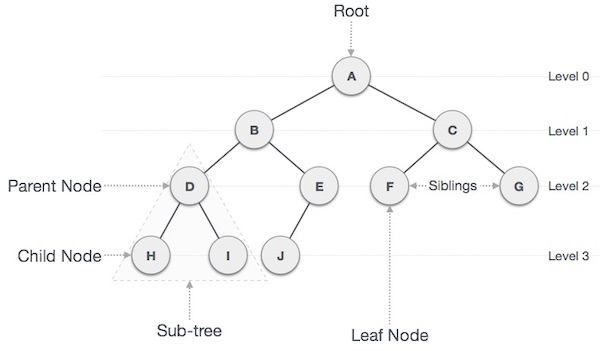The following terms are common with respect to binary trees:

• Path: the sequence of nodes along the edges of a tree. Ex: A-B-D-H or A-B-E-J or A-C-J is a path.
• Root: the top node of a tree. There is only one root and one path from the root to any node of a tree
• Parent: a node that has at least a sub-node
• Child: a sub-node of a node
• Leaf: a node that has no child
• Sub-tree: a sub-tree of a node is a tree that has all descendants of that node. Ex: D-H-I is the sub-tree of node B
• Visiting: checking the value of a node when the pointer is on the node
• Traversal: going through nodes in a specific order
• Level: the generations of nodes. Root node is at the level 0, its child nodes are at the level 1, and its grandchild nodes are at the level 2, and so on
• Key: the value of a node
• Lowest common ancestor (LCA): LCA of node A and node B is the closet parent of BOTH. If node A is the parent of node B so LCA of A and B is A. For example, in the following figure, B is the LCA of A and C.The following code is an example of JavaScript Binary Tree declaration

```function TreeNode(val = 0, left = null, right = null) {
this.val = val;
this.left = left;
this.right = right;
}```

### Types

Here are some example of different types of binary trees:

Note: if a tree is has only the root or has no any node, it could be considered any types below

#### Symmetrical Binary Trees

A symmetrical binary tree is a mirror of itself. In other words, nodes' values are symmetrically equal around the center of the tree).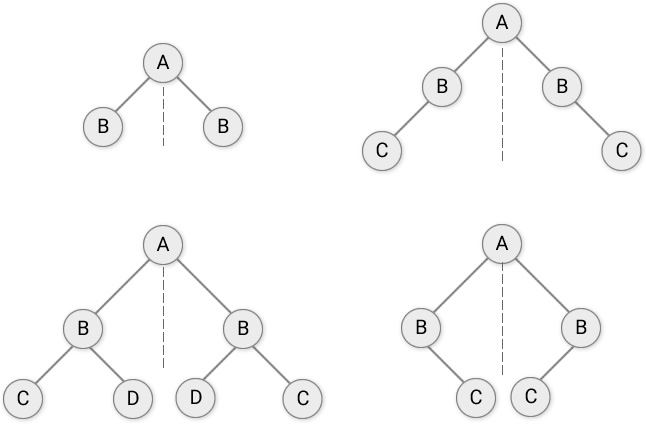#### Full Binary Trees

A full Binary tree (or a proper binary tree) is a special type of binary tree in which every parent node/internal node has either two or no children.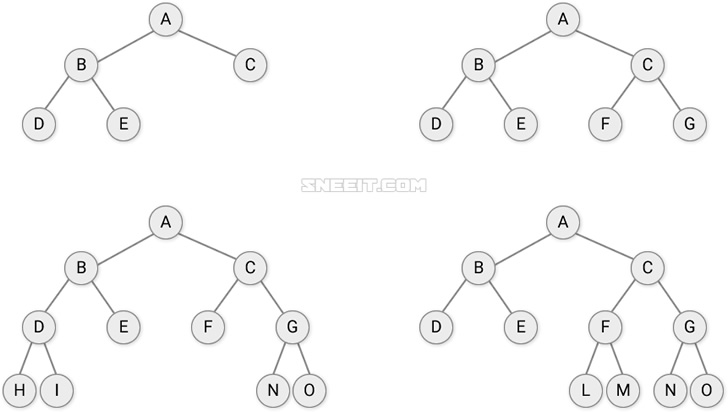#### Complete Binary Trees

A complete binary tree is a binary tree in which all the levels are completely filled except possibly the lowest one, which is filled from the left.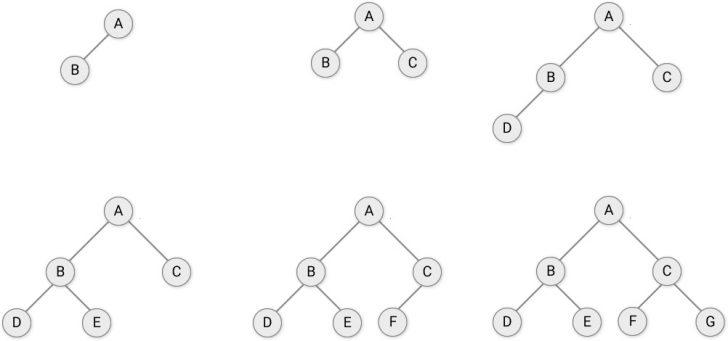#### Perfect Binary Trees

perfect binary tree is a binary tree in which all interior nodes have two children and all leaves have the same depth or same levelNote: some people call complete binary trees as almost complete binary trees and perfect binary trees as complete tree.

#### Binary Search Trees

A binary search tree (BST) is a binary tree in which any given node is always larger than all its left-nodes and smaller than all its right-nodes.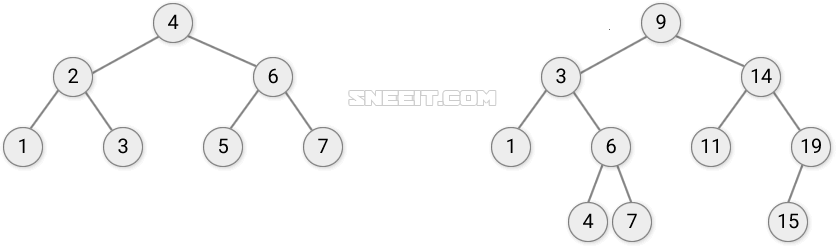In a threaded binary tree,  we point null children of nodes to other nodes of the tree to create advantages for traversal.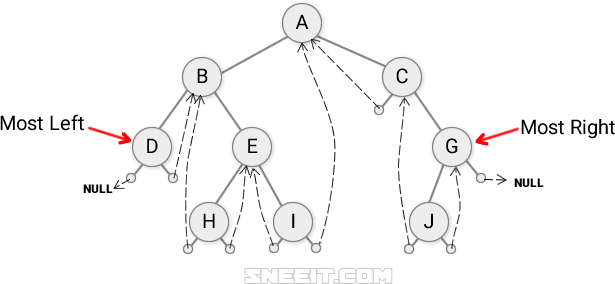#### Heaps

A heap is a complete binary tree in which any given nodes is:

• always greater than or equal to its child nodes => the root node has the max value
=> This tree has MAX HEAP property
• always smaller than equal to child nodes => the root node has the min value
=> This tree has MIN HEAP property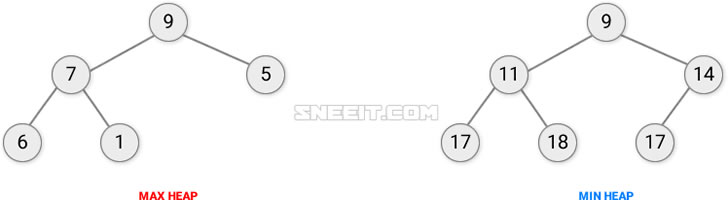## Algorithms

### Traversal

If we have the following binary treeThe most common way to access all nodes is using the below pathHowever, depending on the order of processing values of the nodes, we will have the following traversal orders:

• Pre-order: process the values of the root first, then the left sub-trees and finally the right sub-trees (Root => Left sub-trees => Right sub-trees). The same process will be applied for the sub-trees. For the example tree, here is the process order of the values: F B A D C E G I H
• In-order: Left Sub-trees => Root => Right sub-trees. A B C D E F G H I
• Post order: Left sub-trees => Right sub-trees => Root. A C E D B H I G F
• Level order: traverse level by level or Breath-First Search (BFS). F B G A D I C E H

Post order is widely used, like in deleting nodes when you have to delete all left and right nodes of a node before delete the node itself or in calculating mathematics expressions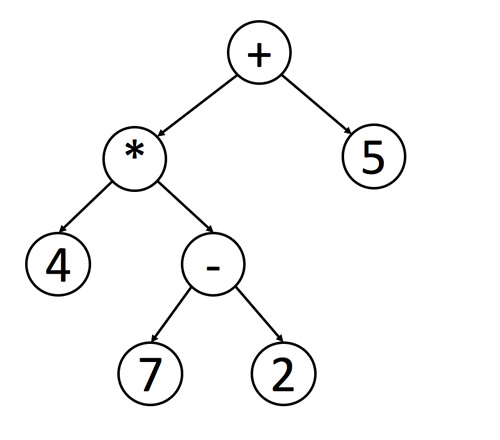You can use recursion or iteration + stack/queue to decide the order of the values that you want to process. Here are some problems related to traversal methods:

Because recursion is the most popular and intuitive algorithm to traverse along binary trees, so I would like to summarize Javascript Codes for Pre-order, In-order and Post-order traversal here:

```// Binary Tree Traversal
var Traversal = function(root) {
let traverse = node => {
// stop condition
if (null == node) return [];

// PRE-ORDER (root-left-right)
//process the current node value
// ....
// then process the left sub-tree
traverse(node.left);
// and the right sub-tree
traverse(node.right);

// IN-ORDER (left-root-right)
/*
traverse(node.left);
traverse(node.right);
*/

// POST-ORDER (left-right-root)
/*
traverse(node.left);
traverse(node.right);
*/
}

// start the traversal
traverse(root);

// final process
// ...
};```

You can practice with some following problems:

## Binary Search Tree (BST)

A binary search tree (BST) is a binary tree in which any given node is always larger than all its left-nodes and smaller than all its right-nodes.### BST Search

Due to the special structure of a BST:

• If the target is equal to the current node's value, return true
• If the target is smaller than the current node's value, so it should be in the left subtree, just traverse to the left sub-tree to find.
• If the target is larger than the current node's value, so it should be in the right sub-tree, just traverse to the right sub-tree to find.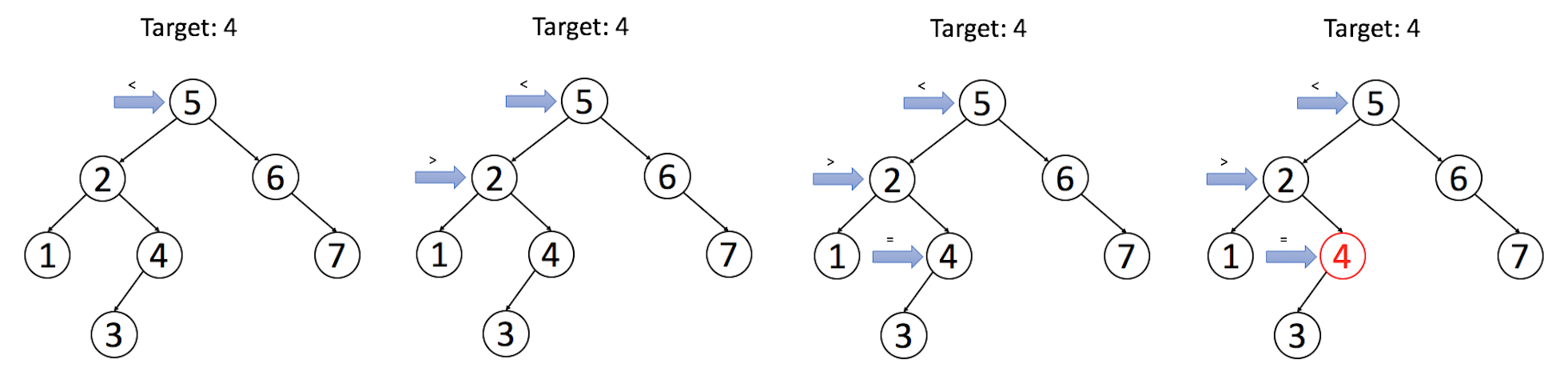Now, let's search in a BST (with Explanation).

### BST Insertion

It clear to see at a node, the target could be:

• Smaller than the node's value, so the target should be in the left sub-tree of the node.
• If the left sub-tree of the node is NULL, the new node with the value of the target will be that sub tree
• Other wise, traverse to the left sub-tree to continue our comparison
• Larger than the node's value, so the target should be in the right sub-tree of the node.
• If the right sub-tree of the node is NULL, the new node with the value of the target will be that sub tree
• Other wise, traverse to the right sub-tree to continue our comparison

In other words, the new node with the target value will always be a leaf node.

Now, let's insert a value into a BST (with Solution)

### BST Deletion

At first, we need to search the node we want to delete in a BST. We call that node is the target. Then:

• If the target has no child, just delete it because it will not affect the BST fundamental
• If the target has one child, just replace it with the child because the relation between the target's child and the target's parent is still following the BST rules
• If the target has two children, you have two options:
1. replace it with the most left node in its right subtree which is smaller than the whole right subtree but larger than the target so, of course, larger than the whole left sub-tree of the target.
2. replace it with the right child. In this case, we will find the first node in the target's right subtree that has empty left (the most left node) to relocate the target's left-subtree into that free space.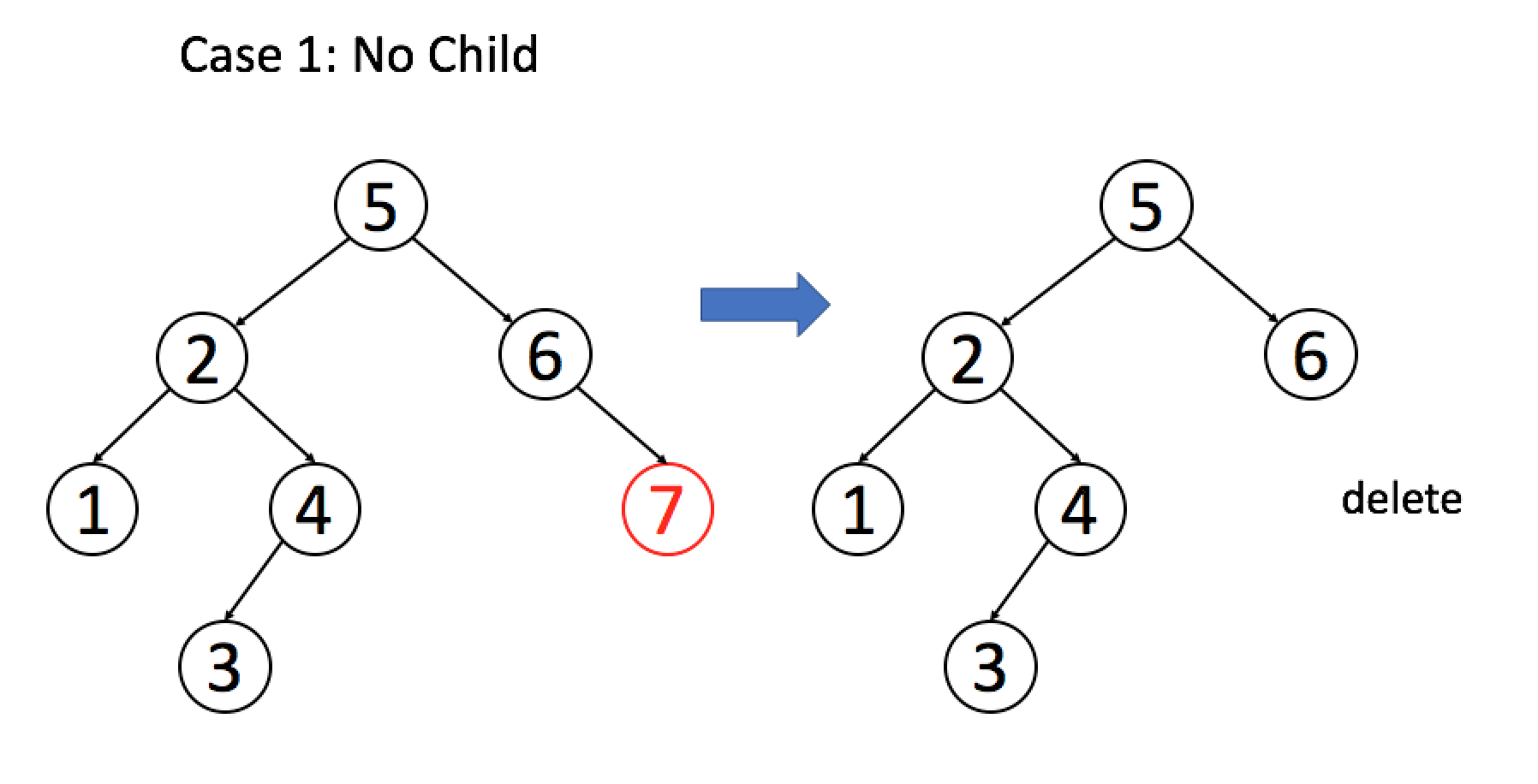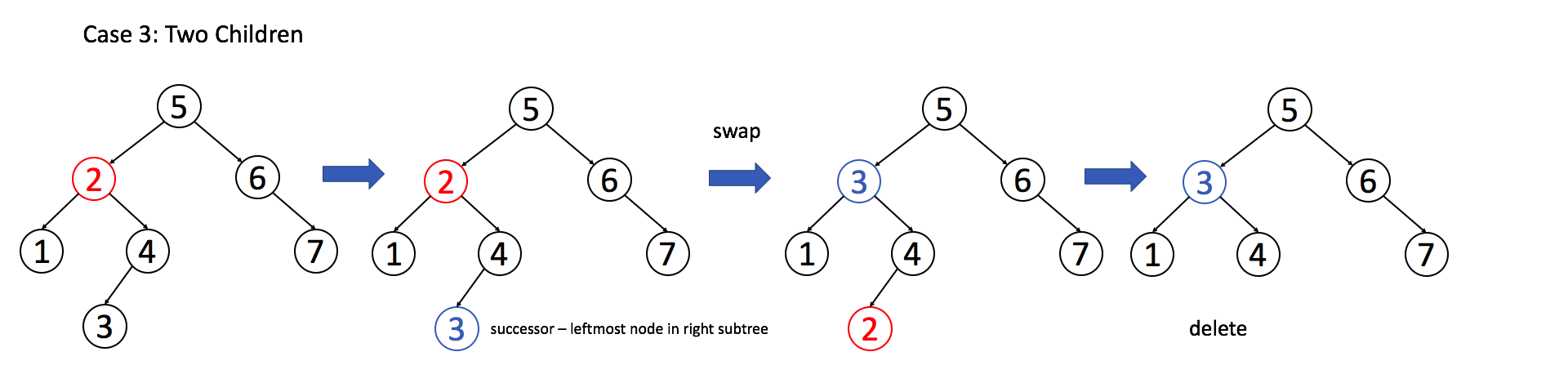Let practice "Delete Node in a BST" problem with EXPLANATION.

### BST Practice

It does not always need BST in the problem description to use BST to solve. Sometimes, if you want to store data in order and need several actions like search, insertion, deletion ... , a BST  might be a good choice because all those operations can be done in O(h) time complexity.

Let's solve "Design a class to find the kth largest element in a stream" problem (with Explanation) to understand about the BST. You can also practice more with:

## Heaps

A heap is a complete binary tree in which any given nodes is:

• always greater than or equal to its child nodes => the root node has the max value
=> This tree has MAX HEAP property
• always smaller than or equal to its child nodes => the root node has the min value
=> This tree has MIN HEAP property### Heap Insertion

Two steps:

1. Insert node right next to the most bottom right child to keep the tree as a complete tree
2. Swap the node with its parents until it satisfies the heap rules (max: parents >= children, or min: parents <= children)Insert 5 into a Min Heap

### Heap Deletion

Two steps:

1. Swap the most bottom right node with the current node that we want to delete then delete the most bottom right node
2. Swap the current node with its smallest child until it satisfies the heap rule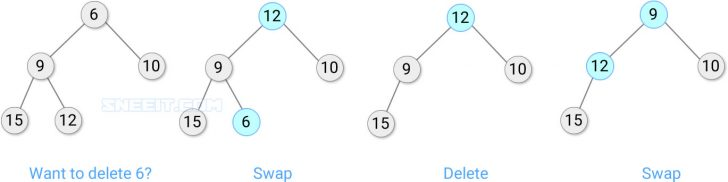Delete 6 from a Min Heap

### Heap to Array

We can store any complete binary tree with N nodes into an array with N+1 elements, following in-level traversal. Then if we are given a node at index I, we could know:

• The parent node is at index I/2
• The left node is at index I*2
• The right node is at index I*2

And all nodes has indexes that larger than N/2 is a leaf node.

Btw, all nodes in Level X will have 2^(X+1) > Index >= 2^X. Min level = 0, Max level =  log2(N).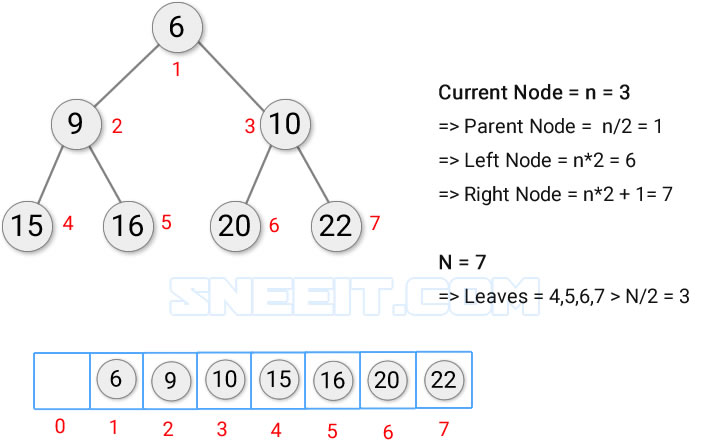Transform a Min Heap to an Array

The array is usually used to save the N (total number of nodes). But if you want to start saving nodes from index 0 (zero-based, ex: heapq in PyThon), then if we are given a node at index I, we could know:

• The parent node is at index (I-1)/2
• The left node is at index I*2+1
• The right node is at index I*2+2

And all nodes has indexes that larger than (N/2-1) is a leaf node.Transform a Min-Heap to an Array Zero-based

Btw, all nodes in Level X will have 2^(X + 1) - 1 > Index >= 2^X - 1. Min level = 0, Max level =  log2(N).

You can use the same logic to transform an array to a complete binary tree.

And with this logic, you can use an array as a heap and perform any heap algorithms like a Heap Tree.

### Heap Construct

To construct a Heap from an array, we need:

1. Convert the array to a Complete Binary Tree
2. Heapify the tree by scanning all nodes from the bottom level to top level. Then if a node is not satisfied the Heap rule, we swap it with its child
• Min Heap: swap with smallest child
• Max Heap: swap with biggest child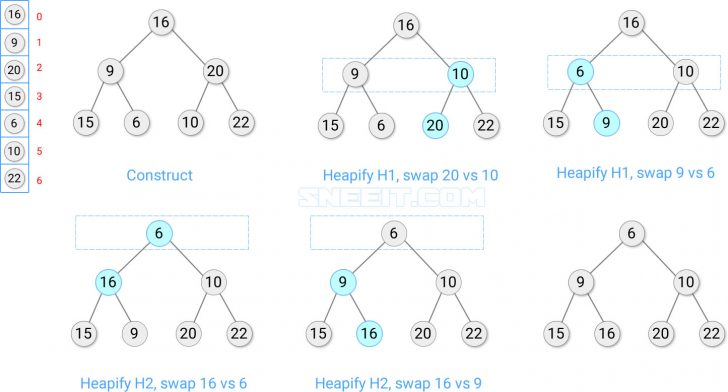Heapify to have a Min Heap

*Note: In PyThon, built-in heap construct function is only for Min Heap, so if you want to construct a Max Heap from an array, you need to multiply all elements in the array with -1. Then after constructing a Min Heap from the array, we multiply all nodes with -1 to convert the Min Heap to a Max Heap

### Time and Space Complexity

The below list is time and space complexity respectively of Heap functions with N is the number of nodes:

• Construct: O(NlogN) O(N)
• Insert: O(logN) O(1)
• Get the top: O(1) O(1)
• Delete the top: O(logN) O(1)
• Get the size: O(1) O(1)
• Heapify a Node: O(logN) O(1)
• Heapify whole Heap: O(NlogN) O(1)

### Heap Sort

If you want to get nodes of a Heap in a certain order, you can:

1. Pick the top node, then swap the last node (most bottom right) to the top node and delete the last node
2. Heapify the top level
3. Repeat from step 1 until the whole tree is empty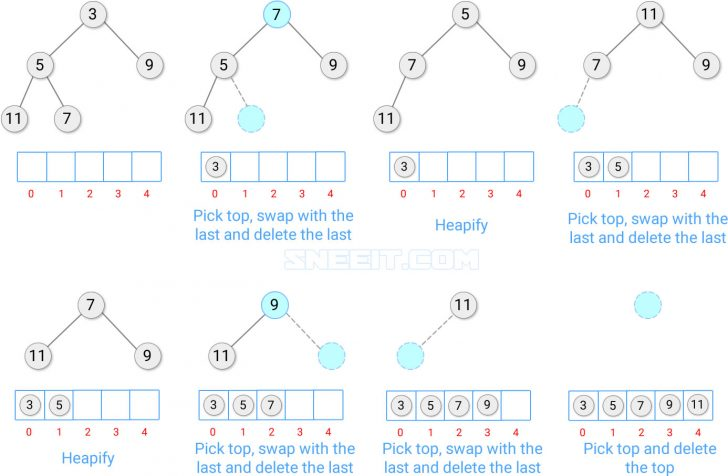Min Heap Sort

### Heap Top K Problem

Using the sort algorithm, it's really easy to find the K smallest elements in a Min Heap or K largest elements in a Max Heap just by doing the sort algorithm until you get enough number of elements you need. If you can't to find the K smallest elements in a Max Heap or K largest elements in a Min Heap, just multiply every elements with -1 and do the sort algorithm to find like above.

With the same logic, we can find the K-th smallest or largest in a Heap.

However, if you are given an array and you don't want to build a full heap and using sort algorithm to find K smallest / largest elements or K-th smallest / largest element, you can:

1. Create an empty binary tree
2. Put first K elements to the tree to build a complete binary tree
3. Heapify to have
• a Max Heap for smallest elements problem
• a Min Heap for largest elements problem
4. From element K+1 to N, compare one by one with the top
• Max Heap: replace the root node with current element if the element > the root node
• Min Heap: replace the root node with current element if the element < the root node
5. Repeat from step 3 until the array is empty. Then all nodes in the Heap is the K elements we want or the root is the K-th element we want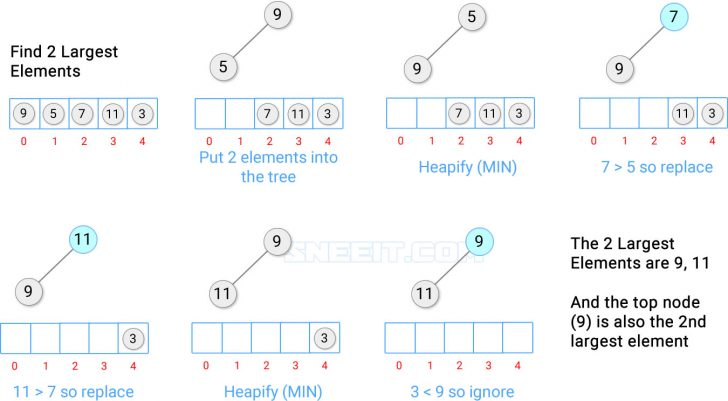Find K largest Elements / K-th Largest Element using a Min Heap